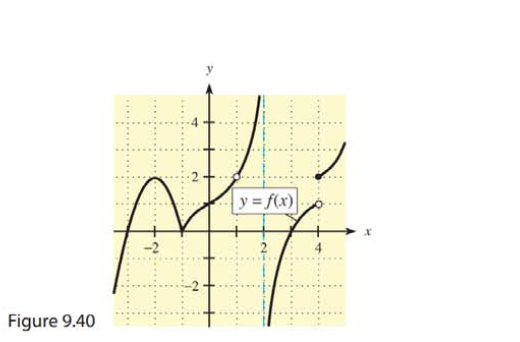Chapter 9, Problem 4REMathematical Applications for the ...

12th Edition
Ronald J. Harshbarger + 1 other
ISBN: 9781337625340

Solutions

Chapter
SectionMathematical Applications for the ...

12th Edition
Ronald J. Harshbarger + 1 other
ISBN: 9781337625340
Textbook Problem

In Problems 1-6, use the graph of y   =   f ( x ) in Figure 9.40 to find the functional values and limits, if they exist.4.   ( a )   lim x → 4 + f ( x )          ( b ) lim x → 4 f ( x )

(a)

To determine

The value of limx4+f(x) by using the provided graph.

Explanation

Given Information:

The graph of y=f(x) is shown below,

The limit is limx4+f(x).

Explanation:

Consider the provided graph,

(b)

To determine

The value of limx4f(x) by using the provided graph.

Still sussing out bartleby?

Check out a sample textbook solution.

See a sample solution

The Solution to Your Study Problems

Bartleby provides explanations to thousands of textbook problems written by our experts, many with advanced degrees!

Get Started

In Exercises 1728, use the logarithm identities to obtain the missing quantity.

Finite Mathematics and Applied Calculus (MindTap Course List)

Prove the statement using the precise definition of a limit. limx2(x23x)=2

Single Variable Calculus: Early Transcendentals, Volume I

Evaluate dx using the Fundamental Theorem of Calculus. a) 7 b) 9 c) d) 18

Study Guide for Stewart's Single Variable Calculus: Early Transcendentals, 8th